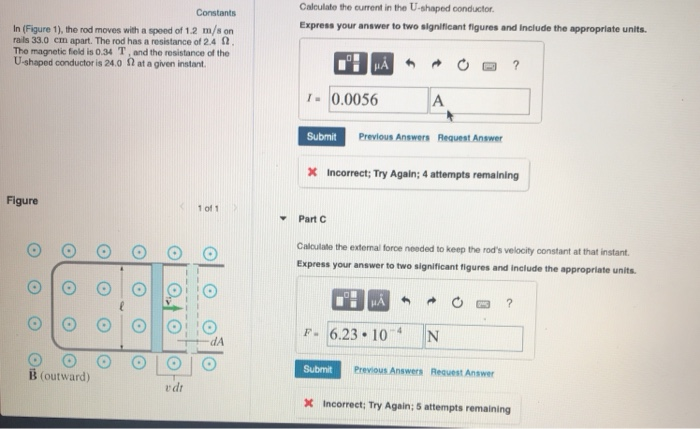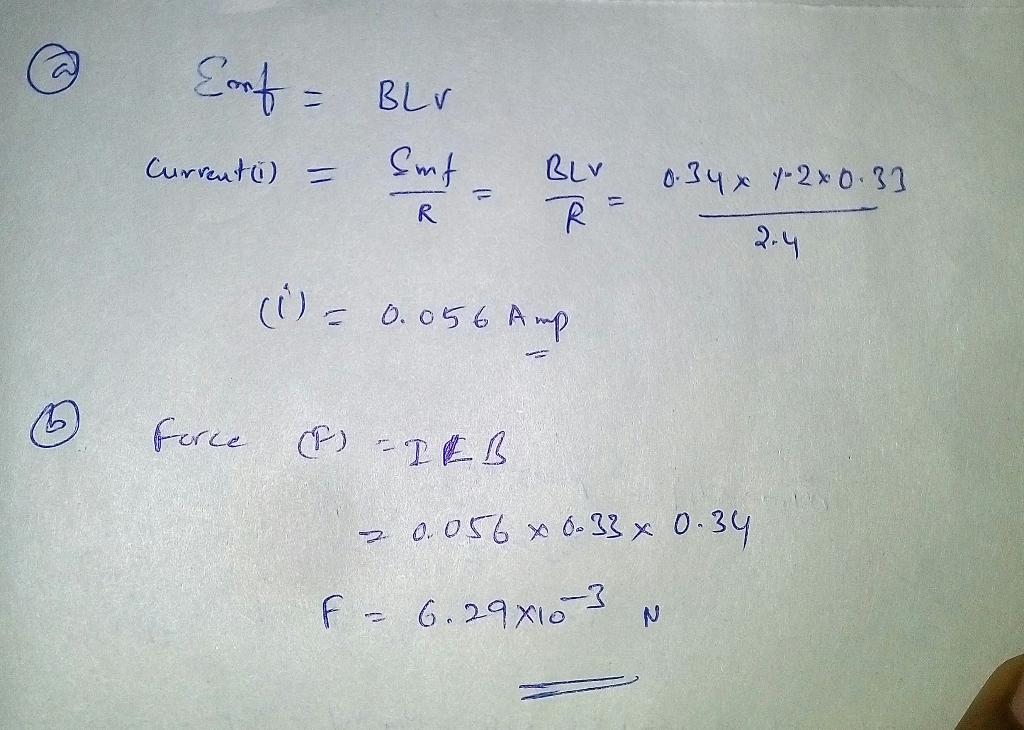Homework Help Question & Answers

# Calculate the current in the U-shaped conductor Express your answer to two significant figures an...Calculate the current in the U-shaped conductor Express your answer to two significant figures and Include the appropriate units Constants In (Figure 1), the rod moves with a speed of 1.2 m/s on rals 33.0 cm apart. The rod has a resistance of 2.4 Ω The magnetic fiold is 0.34 T, and the resistance of the U-shaped conductor is 24.0 Ω at a given instant. 0.0056 Submit Previous Answers Request Answer X incorrect; Try Again; 4 attempts remaining Figure 1 of 1 Part C Caloulate the extenal force needed to keep the rod's velocity constant at that instant. Express your answer to two significant figures and include the appropriate units F 6.23. 10 Submit Previous Answers Request Answer B (outward) u dt Incorrect: Try Again; 5 attempts remaining##### Add Answer of: Calculate the current in the U-shaped conductor Express your answer to two significant figures an...
More Homework Help Questions Additional questions in this topic.

• #### Course Home KHW 32 Problem 10.26 Part A Determine the moment of inertia of the Express your answer to three significant figures and include the appropriate u Take that a 1.5 in. Figure 1 of 1 , 1...

Need Online Homework Help?

Get FREE EXPERT Answers
WITHIN MINUTES
Related Questions